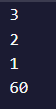# Struggling with Recursion

Tell us what’s happening:
Describe your issue in detail here.
This statement; However, notice that multiply(arr, n) == multiply(arr, n - 1) * arr[n - 1]
is really blowing my mind. I can’t wrap my head around what this is telling me. Can someone break this down and explain it to me like I’m 5? Thanks!

``````
function multiply(arr, n) {
let product = 1;
for (let i = 0; i < n; i++) {
product *= arr[i];
}
return product;
}
``````

However, notice that multiply(arr, n) == multiply(arr, n - 1) * arr[n - 1]

``````  function multiply(arr, n) {
if (n <= 0) {
return 1;
} else {
return multiply(arr, n - 1) * arr[n - 1];
}
}
``````
``````  **Your code so far**
``````
``````
function sum(arr, n) {
// Only change code below this line

// Only change code above this line
}
``````
``````  **Your browser information:**
``````

User Agent is: `Mozilla/5.0 (Windows NT 10.0; Win64; x64) AppleWebKit/537.36 (KHTML, like Gecko) Chrome/97.0.4692.99 Safari/537.36`

Challenge: Replace Loops using Recursion

I’ll tr to explain it in a simple way.

You need to find a key you kept in a box long time ago.
You open a box to find out that there is another box inside it. When you open that one, you find yet another one it in.
This repeats x number of times. You dont know which one has the key.

Lets write a program for this.

``````function openBox(box){
return box;
} else{
openBox(box.open());
}
}
``````

As you can see, this function keeps on opening the box till the key is found.

``````function openBox(box){
return box;
}
``````

What the above code does is → if the box has the key , it will return the value of the box containing the key and the code stops executing.

``````else{
openBox(box.open());
}
}
``````

Else, the function keeps opening the next box until the value in the if() block is met, that is, till the key is found
As you can see, the function calls itself, but it changes its value passed into it each time. In this case , the functions tells itself to open the next box.

This is exactly what recursions do ! They keep on executing until the value passed into the if() block is met. Otherwise, in the else block it will call the function but with a modified value so that it can check if the modified value can pass the code in the if statement.
This is a very simple explanation of recursion imo.

The above was something I tried explaining to someone long ago.

What this piece of code does is multiply the first n elements of an array.
Example `multiply([2, 3, 4, 5], 3)` would return `24` since `2 x 3 x 4` returns 24.
The `n-1` helps give the correct index of the array. Remember that the index starts at 0.

SO each time this would become `multiply([2, 3, 4, 5], 2) * arr` ,
`multiply([2,3,4,5],1) * arr`

Lets take another example. `multiply([2,3,4,5], 3)`.
If you put a `console.log(n)` statement before the return we get this.3, 2, 1 are the values of n .
Here, 60 is the value of `multiply([2, 5 ,6 ], 3)`

That means `console.log(n-1)` would give the values

``````2
1
0
``````

Notice how it stops at 0.

The function multiplies the current value with the previous value and then returns the answer when the condition in the `if()` block is met. So here, this would multiply the returned values until `n=0`.

Loops are actually easier than recursions. You might only really need them for interviews or maybe rarely. Imo, you can do pretty much anything recursion does with loops. But its always good to know how something works .

Hope this basic explanation helped.

1 Like

Hey, you really helped me start to understand recursion, so thank you.

The only part of your explanation that I’m having trouble understanding is

I’m not understanding where the [2, 5, 6] came from as 6 isn’t in the original array you provided as an example, so I’m unsure of how 60 came about as well.

Thanks!

No worries. That was actually an example I tried myself.

Instead of using `multiply([2,3,4,5], 3)` I used `multiply([2, 5, 6], 3)` just to play around with the results. If the first 3 terms are multiplied then the result would be 60. {`2 x 5 x 6 = 60`}.

1 Like

This topic was automatically closed 182 days after the last reply. New replies are no longer allowed.